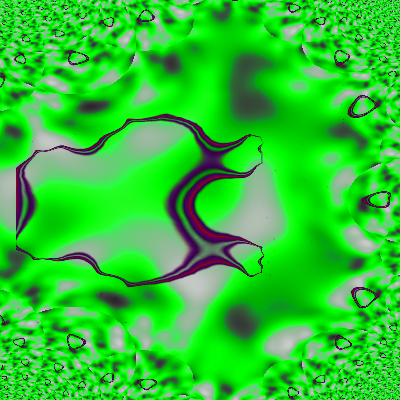# fractal mark

i call it fraclius.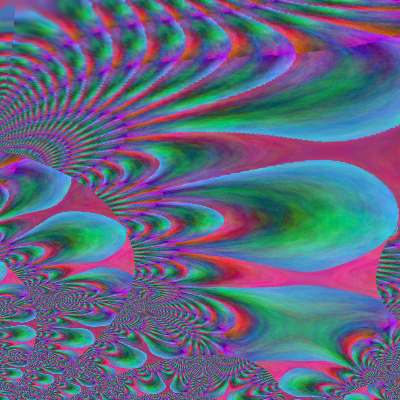i made it with gimp filters=render=clouds=plasma
filters=map=fractal trance

did you read what is at the bottom of the image?

fractal planet.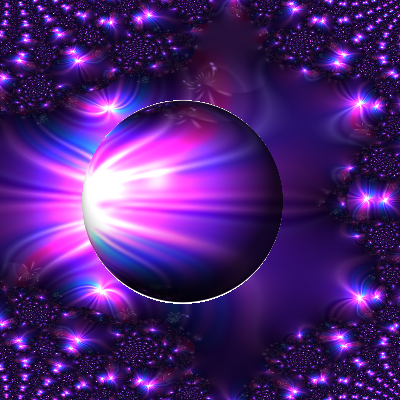this is called fractal light.this is called green greener.this called pink skin fractal.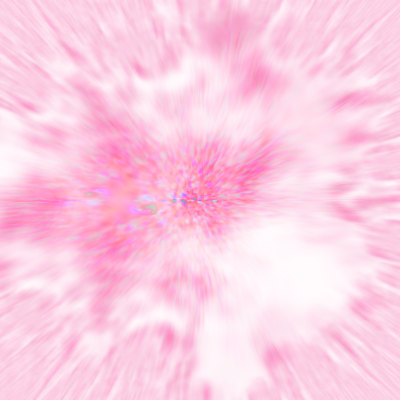this is called fractal mass.this is called black fractal spheres.here is another one called green blood fractal.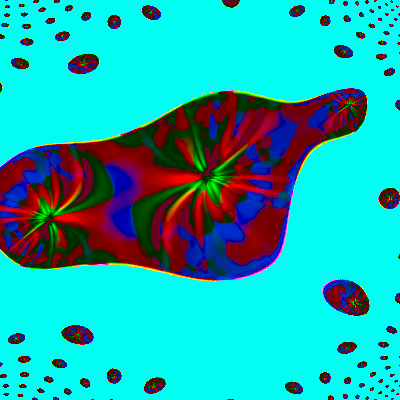here is another one called fractal group.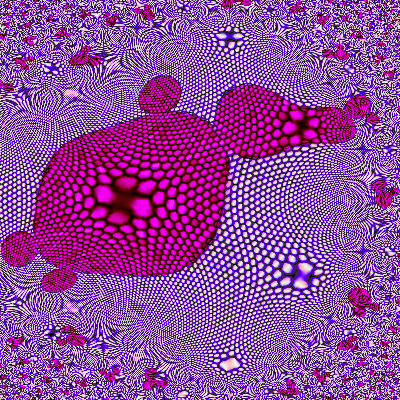here is another one called infinite circle fractals.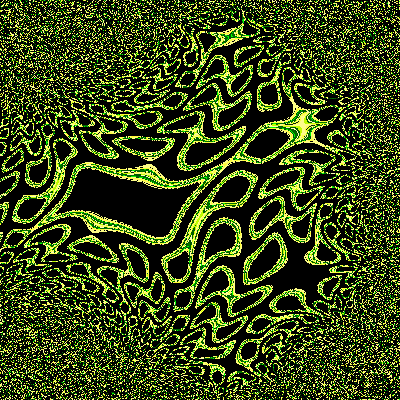here is another one I call fractal lizard skin.here is another fractal did with gimp lava black speck fractals.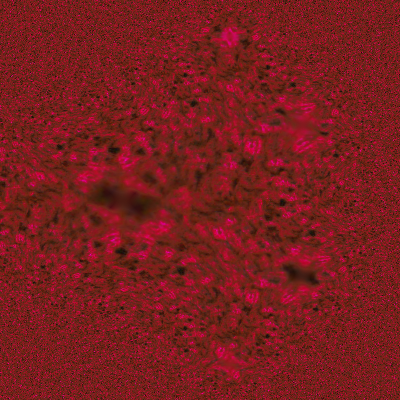here is another fractal I did with gimp called creamy wind.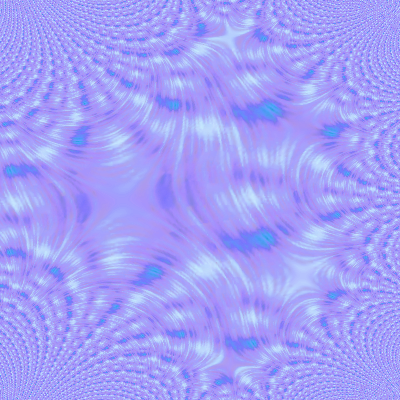here is another fractal I did with gimp called extremely weird fractal.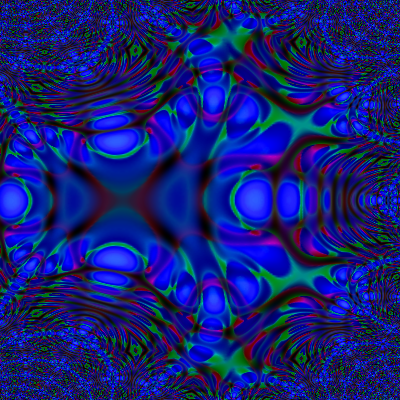here is another fractal I did with gimp called rainbow fractal in the dark.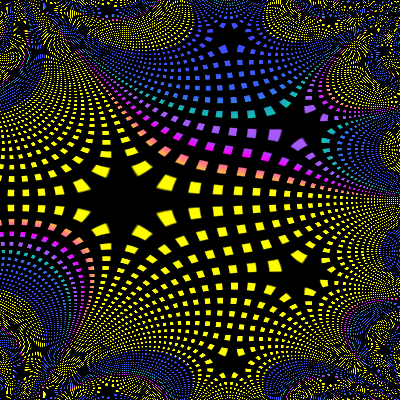here is another fractal I did with gimp called sun sphere fractalhere is another fractal I did with gimp named tornado box fractal.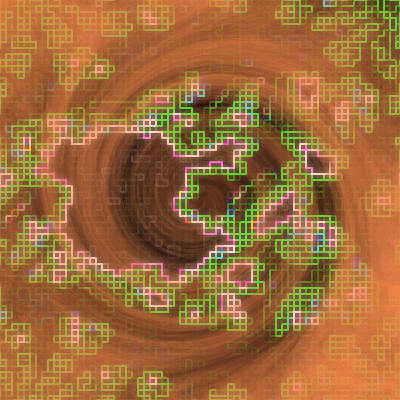here is another fractal i made with gimp named graudius cloud.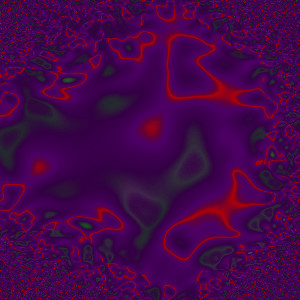here is another fractal i made with gimp named two headed fractal